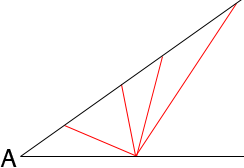SEARCH HOMEMath Central Quandaries & QueriesQuestion from Allen, a student: How to find the other two angles of a scalene triangle if one angle is givenWe have two responses for you

Hi Allen,

If you only know the measure of one angle of a triangle then there are many choices for the measure of the other two angles. The only requirement is that the sum of the measures of the three angles must be 180 degrees.Penny

Hi Allen.

For a scalene triangle you need to know more information than just one angle.

Imagine any scalene triangle and consider any one angle. Could you not
lengthen or shorten the two sides joining that angle and create a different
scalene triangle, preserving the one angle, but causing the other two
angles to change?

A scalene triangle, like any triangle, has angles adding up to 180 degrees so
if you know that one angle is, for example, 40 degrees, the only thing you
can say without knowing more about the triangle is that the other two angles
total 140 degrees.

Cheers,
Stephen La RocqueMath Central is supported by the University of Regina and The Pacific Institute for the Mathematical Sciences.